# NCERT solutions class 9 maths linear equations in two variables 4.4

In this page we have NCERT book Solutions for Class 9th Maths Linear equations in two variables for EXERCISE 4.4 on page 77. Hope you like them and do not forget to like , social share and comment at the end of the page.

## Chapter 4 Ex 4.4

Question 1
Give the geometric representations of y = 3 as an equation
(i) in one variable
(ii) in two variables
Solution
(i) The representation of the y=3 on the number line is shown in below figure where y=3 is treated as an equation in one variable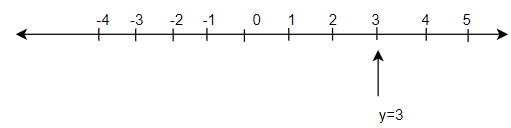(ii) This can be treated as equation in two variable as
$0 \times x + y =3$
Drawing the graph by putting x=0 and x=1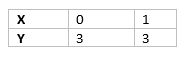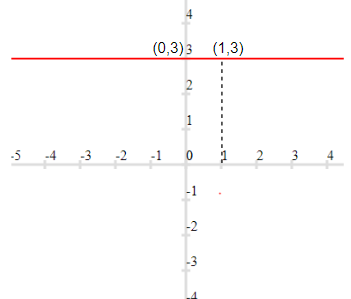So ,it is a line parallel to X-axis

Question 2
Give the geometric representations of 2x + 9 = 0 as an equation
(i) in one variable
(ii) in two variables
Solution
(i) The representation of the $2x+9=0$ on the number line is shown in below figure where x=-9/2 =4.5 is treated as an equation in one variable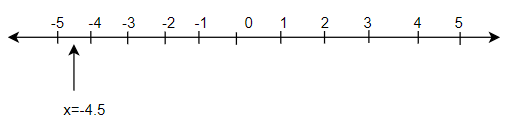(ii) This can be treated as equation in two variable as
$2x + 0 \times y +9=0$
Drawing the graph by putting y=0 and y=2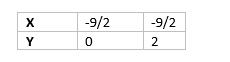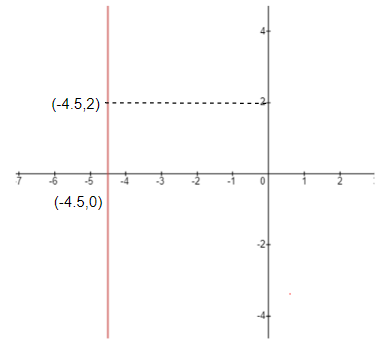So ,it is a line parallel to Y-axis

## Summary

1. NCERT solutions class 9 maths linear equations in two variables 4.4 has been prepared by Expert with utmost care. If you find any mistake.Please do provide feedback on mail. You can download the solutions as PDF in the below Link also
2. This chapter 4 has total 4 Exercise 4.1 ,4.2,4.3 and 4.4. This is the Last exercise in the chapter.You can explore previous exercise of this chapter by clicking the link below